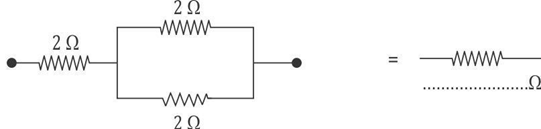SSC BOARD PAPERS IMPORTANT TOPICS COVERED FOR BOARD EXAM 2024

### SSC Boards SCIENCE & TECHNOLOGY QUESTION PAPER FOR THE YEAR 2015.

SSC Boards SCIENCE & TECHNOLOGY QUESTION PAPER FOR THE YEAR 2015.

This paper was mainly based on the contents of the Government prescribed textbook. It is very cleared from the paper that the textbook is a powerful instrument and source in science education and hence to use it effectively by the students is mandatory.

Most of the questions test the students 'knowledge and reasoning ability rather then their capacity in learning by heart. Therefore, students are supposed to know the lessons very well by understanding the terms, facts, concepts, activities, etc. from the textbook clearly.

In March, 2015 Question paper Chapters 4 , 13 had highest marks allotted. Majority of the questions asked were from the textbooks lessons rather than from the expected exercises in the textbook. Some of the questions were twisted or implicit which confused the students are found difficult to attempt. Hence, to attempt HOTS questions the student should be through with the concepts and activities given in the textbook. For objectives the students should prepare for all chapters.

Conclusion: SSC board papers for Science and Technology maintained good standards and students should be well prepared for attempting such papers.

Maharashtra State Board  Class X Science and Technology  Board Paper – 2015

Time: 3 hrs         Max. Marks: 80Note: To Know Detailed view of this paper Please click the link below.

### SSC Boards SCIENCE & TECHNOLOGY QUESTION PAPER FOR THE YEAR 2015.

Use the same answer-sheet for Section A and Section B.
Draw well-labelled diagrams wherever necessary.
All questions are compulsory.
Students should write the answers of questions in sequence.SECTION A

1. (A) (a) Rewrite the following statements with suitable words in the blanks:   

(i) The device used for producing electric current is called _________. (generator)

(ii) _________ the second layer of the atmosphere reaches 48 km above the Earth’s surface. (Stratosphere)

b. Rewrite the following table so as to match the second column with first 

 Column A Column B (i) eosin (1) losing hydrogen (ii) oxidation (2) synthetic indicator (3) losing oxygen (4) natural indicator

Ans.  (i) eosin (2) synthetic indicator
(ii) oxidation (1) losing hydrogen

(c) Give the molecular formula of bleaching powder.       

(B) Rewrite the following statements by selecting the correct options :    

1. When phenolphthalein is added to NaOH, the colour of the solution will become _________. (colourless)

a. colourless

b. red

c. pink

d. yellow

2. If the potential difference across the ends of a conductor is 220 V and the resistance of the conductor is 44 Ω (ohm), then the current flowing through is _________. (5A)

a. 0.2 A

b. 0.5 A

c. 2 A

d. 5 A

3. 1 A = _________ mA  (103)

a. 102

b. 103

c. 10-3

d. 10-6

4. The distance between principal focus and optical centre of the lens is _________. (focal length)

a. diameter

b. focal length

c. principal axis

d. optical centre

5. When rays of light are incident on a glass slab then the incident ray and emergent ray are ________ each other. (parallel)

a. perpendicular

b. parallel

c. opposite

d. concurrent

2. Solve any five of the following:            

2. Complete the following reaction, balance it and write the name of the products CuO + HCI → _________ + _________

Ans. CuO + 2HCl → CuCl2 + H2O
The name of the products are copper chloride (CuCl2) (also known as cupric chloride or copper chloride) and water (H2O).

4. The velocity of light in a medium is 1.5 × 108 m/s. What is the refractive index of the medium with respect to air, if the velocity in air is 3 x 108 m/s?

Solution : Here, v1 = 1.5 × 108 m/s and v2 = 3 x 108 m/s.
We know that, the refractive index with respect to air is
2η1 = v2 ÷ v1 = (3 x 108 ) ÷ (1.5 × 10) = 2
Thus, the refractive index of the medium with respect to air is 2.

3. Answer any five of the following :        

(b)4.  Answer any one of the following :      

SECTION B

5. (A) (a) Find the correlation in the given pair and rewrite the answer :   

(1) Tinning : Tin : : Galvanizing : _________  (Zinc)

(2) Mammals : _________ : : Amphibia : Fishes   (Reptiles)

(b) State True or False :  

1. Solar water – heater works on renewable energy system

True

2. In human beings the blood goes to the heart in one cycle once.

False: In human beings, the blood goes to the heart twice in one cycle. This is called double circulation.

3. In frogs, thyroid secretion stimulates the metamorphosis from tadpole to adult frog.

True.

(B) Rewrite the following statements by selecting the correct options :    

1. The molecular formula of acetic acid is _________ . (CH3COOH)

a. CH3COOH

b. CH3 – CH3

c. C6H6

d. C2H4

2. Carbon dioxide enters into the leaves through tiny pores present on the surface of the leaf called _________. (stomata)

a. chlorophyll

b. chloroplast

c. stomata

d. epidermis

3. _________ solution is blue in colour. (CuSO4)

CuSO4

b. FeSO4

c. ZnSO4

d. Al2(SO4)3

4. Yeast reproduces by _________. (budding)

a. spore formation

b. multiple fission

c. fragmentation

d. budding

5 Raisins put in water absorb water by the process of _________. ( osmosis)

a. diffusion

b. osmosis

c. transpiration

d. excretion

6. Solve any five of the following :           

7. Answer any five of the following :        

8. Answer any one of the following :